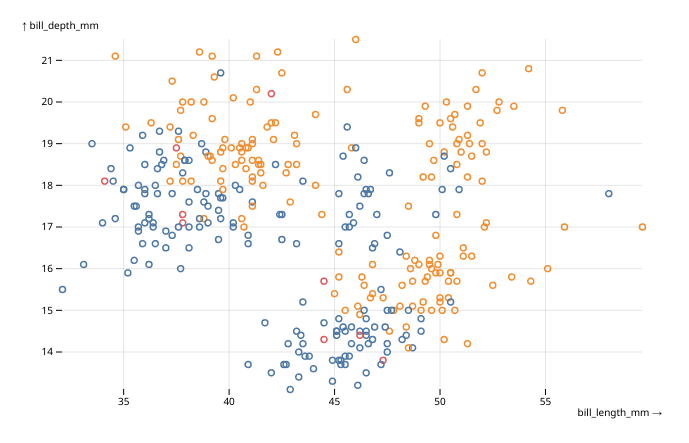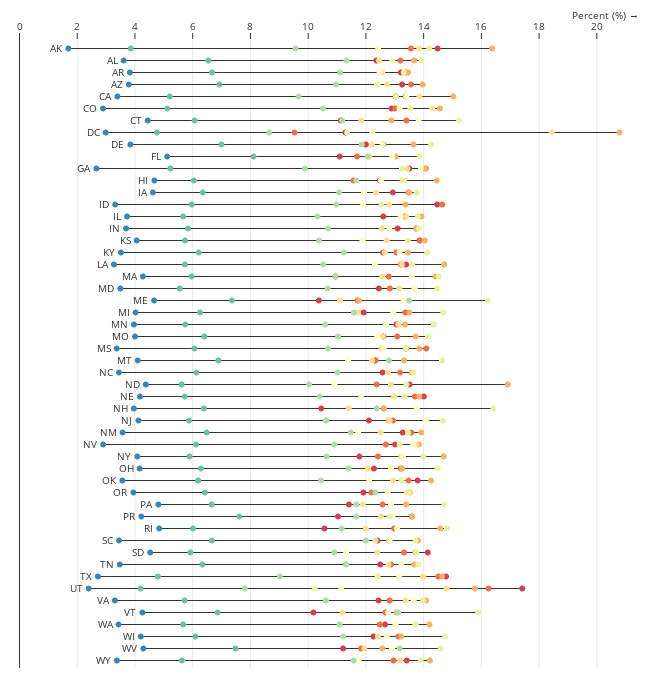obsplot is an experimental attempt at using Observable Plot as HTML widget in R.

Please note that the package is in an early stage. Its API is subject to breaking changes, there are bugs, and maybe bad design choices. But if you still want to try it, issues and feedback are very welcome !

## Examples

Here is a small example of a simple scatterplot from the penguins dataset of the palmerpenguins package :

library(obsplot)
library(palmerpenguins)
data(penguins)

obsplot(penguins) |>
mark_dot(x = bill_length_mm, y = bill_depth_mm, stroke = sex) |>
opts(grid = TRUE)Simple scatterplot

And here is a much more complex example taken from Observable Plot documentation :

data(stateage)

xy <- transform_normalizeX(basis = "sum", z = "name", x = "population", y = "name")

obsplot(stateage, height = 660) |>
mark_ruleX(x = 0) |>
mark_ruleY(
transform_groupY(list(x1 = "min", x2 = "max"), xy)
) |>
mark_dot(xy, fill = age, title = age) |>
mark_text(
transform_selectMinX(xy), textAnchor = "end", dx = -6, text = name
) |>
scale_x(
axis = "top",   label =  "Percent (%) →",
transform = JS("d => d * 100")
) |>
scale_y(axis = NULL) |>
scale_color(scheme = "spectral", domain = unique(stateage\$age)) |>
opts(grid = TRUE)stateage example

You will find many more examples in the two gallery vignettes :

## Usage

To get started, take a look at the introduction vignette.

## Installation

From Github :

remotes::install_github("juba/obsplot")

From R-universe :

install.packages("obsplot", repos = "https://juba.r-universe.dev")

## Development notes

This package uses packer to manage JavaScript source code and dependencies. If you want to modify it, you’ll need a working installation of Node.js.

After cloning this repository, run the following in a terminal at the project root :

npm install

Then, if you modify the JavaScript code in srcjs, you’ll have to run the following command to bundle and update the widget JavaScript code :

packer::bundle_dev()

If you want to ship a minimized production version, use :

packer::bundle_prod()